# 2 Values and types Types Primitive types Composite

• Slides: 482 Values and types § Types § Primitive types § Composite types – cartesian products – disjoint unions – mappings § Recursive types § Type systems § Expressions Programming Languages 3 © 2012 David A Watt, University of Glasgow 2 -1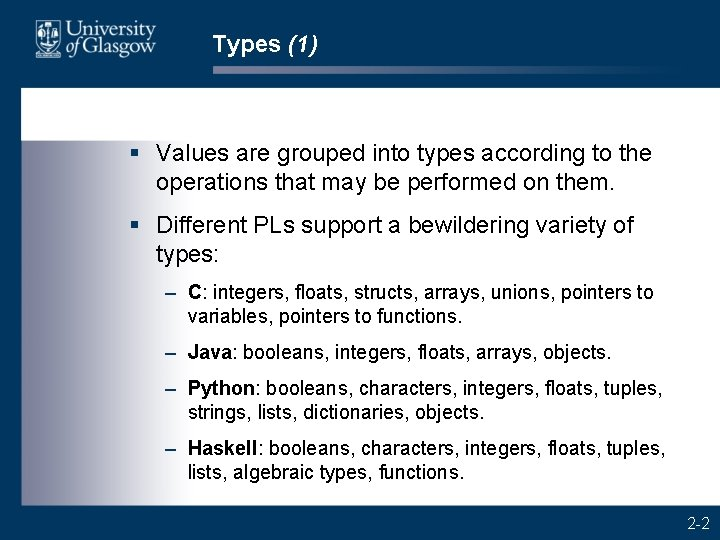Types (1) § Values are grouped into types according to the operations that may be performed on them. § Different PLs support a bewildering variety of types: – C: integers, floats, structs, arrays, unions, pointers to variables, pointers to functions. – Java: booleans, integers, floats, arrays, objects. – Python: booleans, characters, integers, floats, tuples, strings, lists, dictionaries, objects. – Haskell: booleans, characters, integers, floats, tuples, lists, algebraic types, functions. 2 -2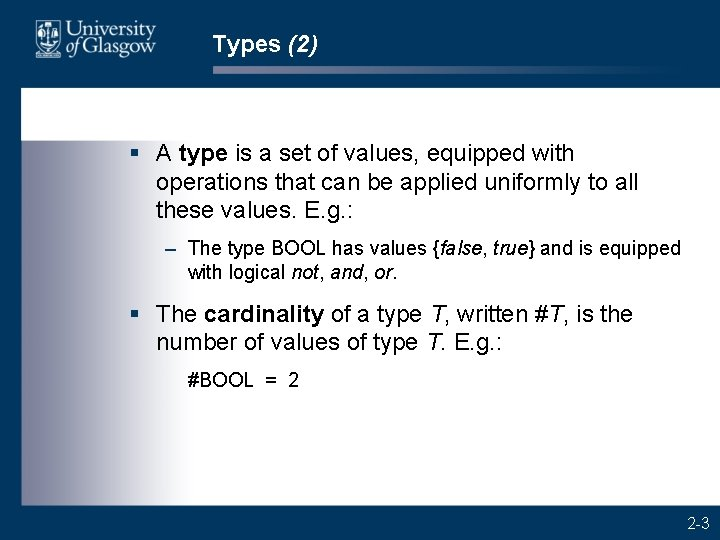Types (2) § A type is a set of values, equipped with operations that can be applied uniformly to all these values. E. g. : – The type BOOL has values {false, true} and is equipped with logical not, and, or. § The cardinality of a type T, written #T, is the number of values of type T. E. g. : #BOOL = 2 2 -3Types (3) § Value v is of type T if v set of values of T. E. g. : – the value false is of type BOOL – the value 13 is of type INT. § Expression E is of type T if, when evaluation of E terminates normally, it is guaranteed to yield a value of type T. E. g. (assuming that variable n is of type INT): – the Java expression “n - 1” is of type INT – the Java expression “n > 0” is of type BOOL. 2 -4Primitive types (1) § A primitive type is a type whose values are primitive (i. e. , cannot be decomposed into simpler values). § Every PL provides built-in primitive types. The choice of primitive types is influenced by the PL’s intended application area, e. g. : – Fortran (scientific computing) has floating-point numbers – Cobol (data processing) has fixed-length strings – C (system programming) has bytes and pointers. § Some PLs also allow programs to define new primitive types. 2 -5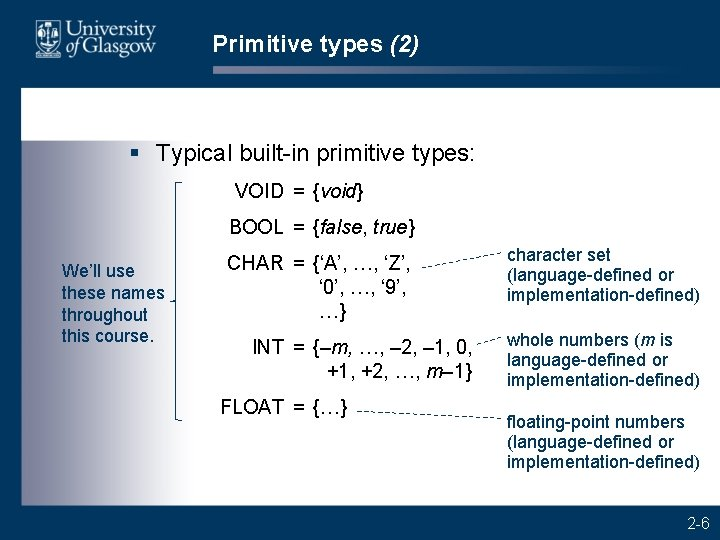Primitive types (2) § Typical built-in primitive types: VOID = {void} BOOL = {false, true} We’ll use these names throughout this course. CHAR = {‘A’, …, ‘Z’, ‘ 0’, …, ‘ 9’, …} INT = {–m, …, – 2, – 1, 0, +1, +2, …, m– 1} FLOAT = {…} character set (language-defined or implementation-defined) whole numbers (m is language-defined or implementation-defined) floating-point numbers (language-defined or implementation-defined) 2 -6Primitive types (3) § Cardinalities: #VOID = 1 #BOOL = 2 #CHAR = 128 or 256 or 32768 #INT = 2 m § Definitions of primitive types vary from one PL to another. – In C, booleans and characters (and enumerands) are just small integers. – In Java, characters are just small integers. 2 -7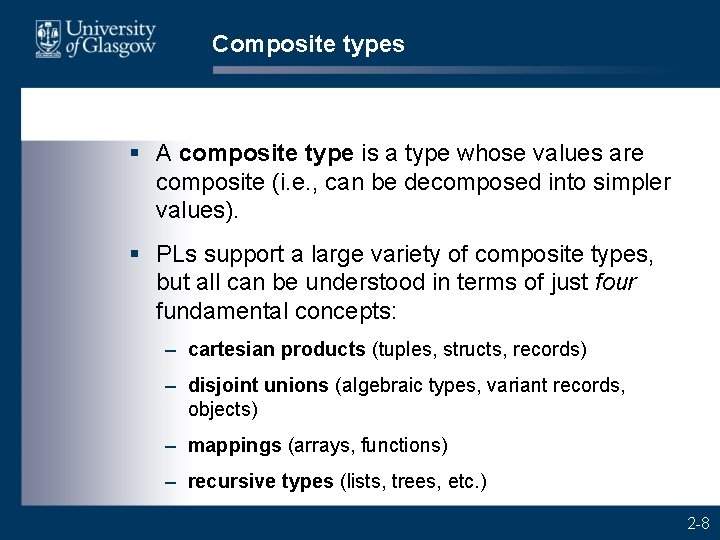Composite types § A composite type is a type whose values are composite (i. e. , can be decomposed into simpler values). § PLs support a large variety of composite types, but all can be understood in terms of just four fundamental concepts: – cartesian products (tuples, structs, records) – disjoint unions (algebraic types, variant records, objects) – mappings (arrays, functions) – recursive types (lists, trees, etc. ) 2 -8Cartesian product types (1) § In a cartesian product, values of two (or more) given types are grouped into pairs (or tuples). § In mathematics, S T is the type of all pairs (x, y) such that x is chosen from type S and y is chosen from type T: S T = { (x, y) | x S; y T } § Cardinality of a cartesian product type: #(S T) = #S #T NB 2 -9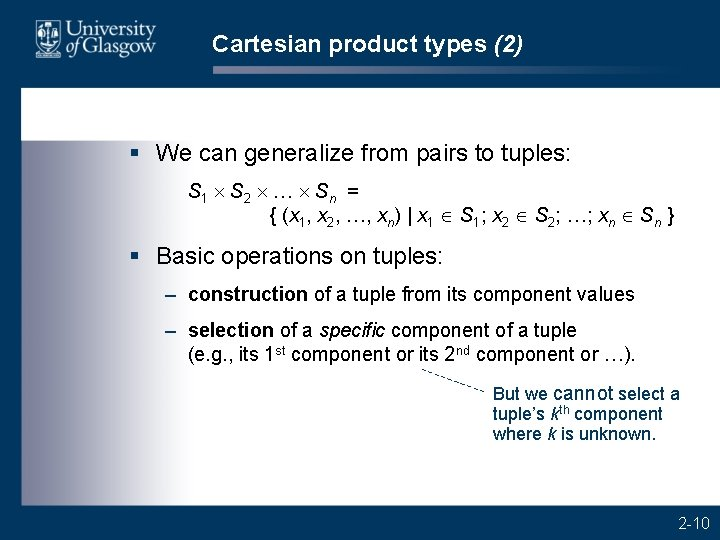Cartesian product types (2) § We can generalize from pairs to tuples: S 1 S 2 Sn = { (x 1, x 2, , xn) | x 1 S 1; x 2 S 2; …; xn Sn } § Basic operations on tuples: – construction of a tuple from its component values – selection of a specific component of a tuple (e. g. , its 1 st component or its 2 nd component or …). But we cannot select a tuple’s kth component where k is unknown. 2 -10Cartesian product types (3) § Pascal records, C structs, and Haskell tuples can all be understood in terms of Cartesian products. § Note that Python’s so-called “tuples” are anomalous. They can be indexed, like arrays. They are not tuples in the mathematical sense. 2 -11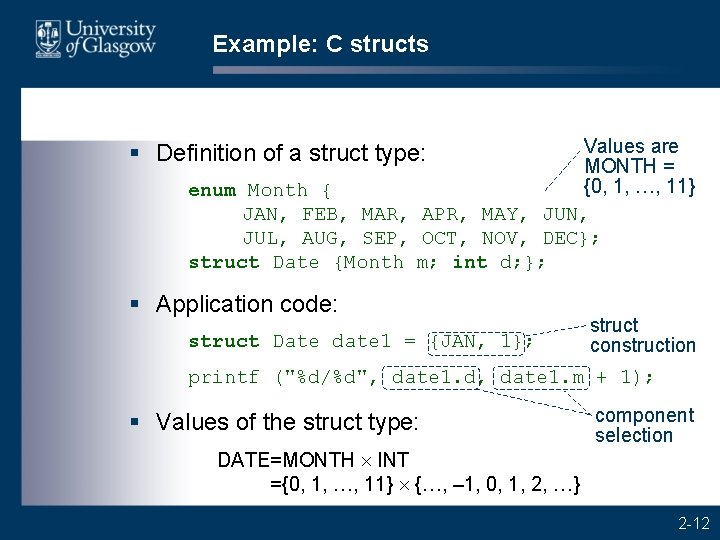Example: C structs Values are MONTH = {0, 1, …, 11} enum Month { JAN, FEB, MAR, APR, MAY, JUN, JUL, AUG, SEP, OCT, NOV, DEC}; struct Date {Month m; int d; }; § Definition of a struct type: § Application code: struct Date date 1 = {JAN, 1}; struct construction printf ("%d/%d", date 1. d, date 1. m + 1); § Values of the struct type: component selection DATE=MONTH INT ={0, 1, …, 11} {…, – 1, 0, 1, 2, …} 2 -12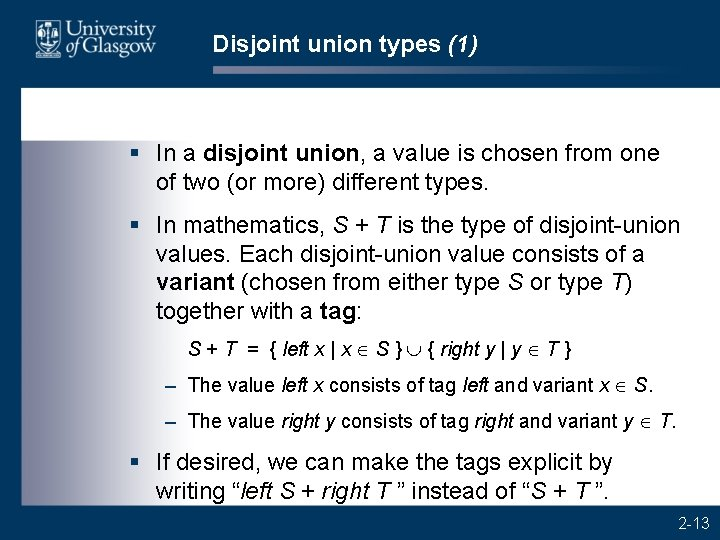Disjoint union types (1) § In a disjoint union, a value is chosen from one of two (or more) different types. § In mathematics, S + T is the type of disjoint-union values. Each disjoint-union value consists of a variant (chosen from either type S or type T) together with a tag: S + T = { left x | x S } È { right y | y T } – The value left x consists of tag left and variant x S. – The value right y consists of tag right and variant y T. § If desired, we can make the tags explicit by writing “left S + right T ” instead of “S + T ”. 2 -13Disjoint union types (2) § Cardinality of a disjoint union type: #(S + T) = #S + #T NB § We can generalize to disjoint unions with multiple variants: T 1 + T 2 + + Tn. § Haskell algebraic types, Pascal/Ada variant records, and Java objects can all be understood in terms of disjoint unions. 2 -14Disjoint union types (3) § Basic operations on disjoint-union values in T 1 + T 2 + + T n: – construction of a disjoint-union value from its tag and variant – tag test, to inspect the disjoint-union value’s tag – projection, to recover a specific variant of a disjointunion value (e. g. , its T 1 variant or its T 2 variant or …). Attempting to recover the wrong variant fails. 2 -15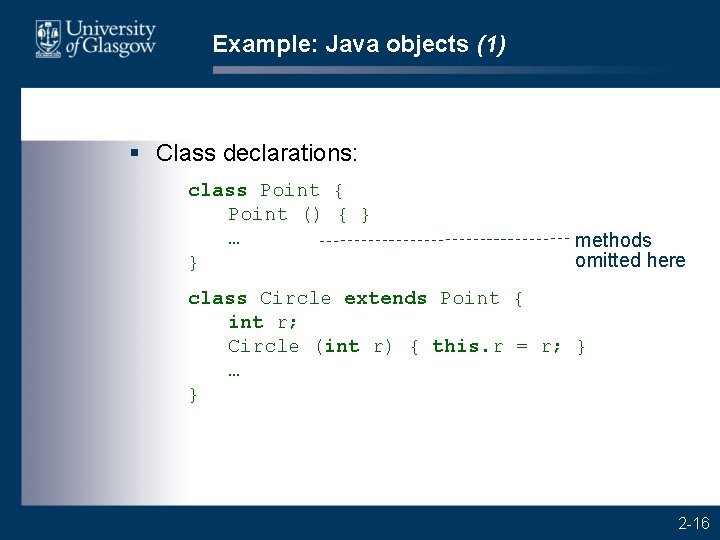Example: Java objects (1) § Class declarations: class Point { Point () { } … } methods omitted here class Circle extends Point { int r; Circle (int r) { this. r = r; } … } 2 -16Example: Java objects (2) § Class declarations (continued): class Box extends Point { int w, h; Box (int w, int h) { … } 2 -17Example: Java objects (3) § Set of objects in this program: OBJECT = … + Point VOID + Circle INT + Box (INT INT) +… § These objects include: objects of library classes objects of other declared classes Point void, Circle 1, Circle 2, Circle 3, …, Box (1, 1), Box (1, 2), Box (2, 1), … § Each object’s tag identifies its class. 2 -18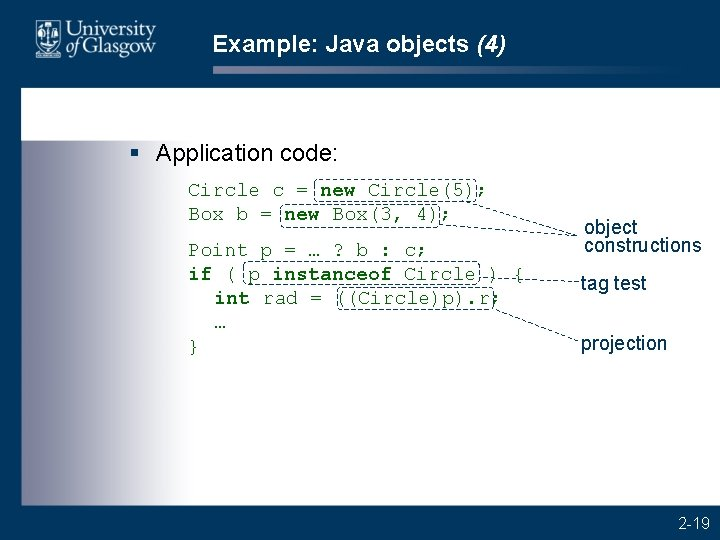Example: Java objects (4) § Application code: Circle c = new Circle(5); Box b = new Box(3, 4); Point p = … ? b : c; if ( p instanceof Circle ) { int rad = ((Circle)p). r; … } object constructions tag test projection 2 -19Example: Java objects (5) § Note that the set of objects in a Java program is open-ended: – Initially the set contains objects of library classes (nonabstract). – Subsequently the set is augmented by each declared class (non-abstract). § Note that abstract classes are excluded. (It is not possible to create an object of an abstract class. ) 2 -20Mapping types § In mathematics, m : S T states that m is a mapping from type S to type T, i. e. , m maps every value in S to some value in T. § If m maps value x to value y, we write y = m(x), and we call y the image of x under m. § S T is the type of all mappings from S to T: S T = { m | x S Þ m(x) T } § Cardinality of a mapping type: #(S T) = (#T)#S since there are #S values in S, and each such value has #T possible images 2 -21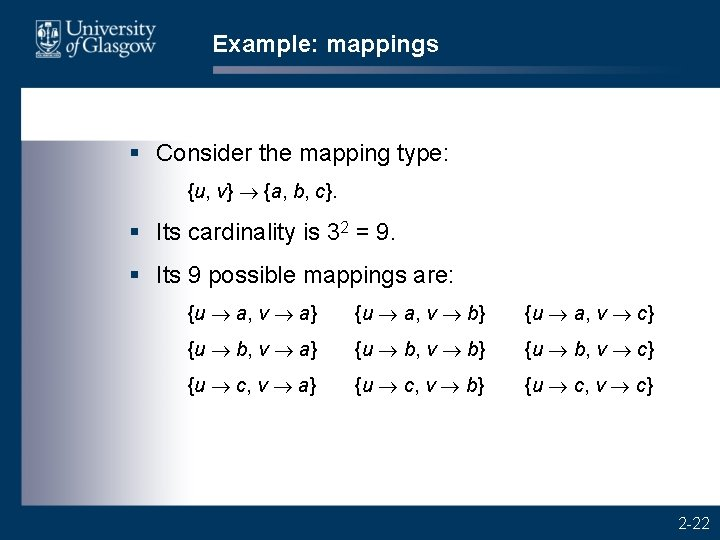Example: mappings § Consider the mapping type: {u, v} {a, b, c}. § Its cardinality is 32 = 9. § Its 9 possible mappings are: {u a, v a} {u a, v b} {u a, v c} {u b, v a} {u b, v b} {u b, v c} {u c, v a} {u c, v b} {u c, v c} 2 -22Array types (1) § Arrays can be understood as mappings. § If an array’s components are of type T and its index values are of type S, the array has one component of type T for each value in type S. Thus the array’s type is S T. § Basic operations on arrays: – construction of an array from its components – indexing, to select a component using a computed index value. We can select an array’s kth component, where k is unknown. (This is unlike a tuple. ) 2 -23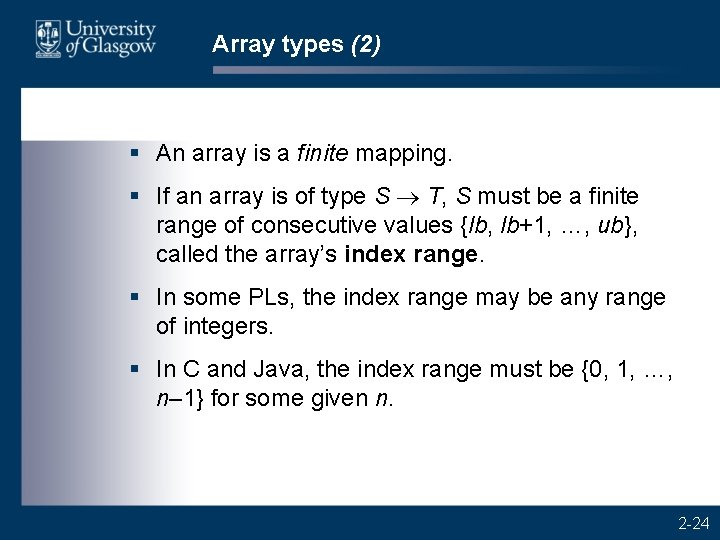Array types (2) § An array is a finite mapping. § If an array is of type S T, S must be a finite range of consecutive values {lb, lb+1, …, ub}, called the array’s index range. § In some PLs, the index range may be any range of integers. § In C and Java, the index range must be {0, 1, …, n– 1} for some given n. 2 -24Example: C arrays (1) § Definition of an array type: enum Pixel {DARK, LIGHT}; typedef Pixel[] Row; Values are PIXEL = {0, 1}. § Application code: Row r = {DARK, LIGHT, DARK}; int i, j; array construction r[i] = r[j]; array indexing § Values of this array type: ROW = {0, 1, 2, …} PIXEL = {0, 1, 2, …} {0, 1} 2 -25Function types § Functions can also be understood as mappings. They map arguments to results. § Consider a unary function f whose argument is of type S and whose result is of type T. Then f ’s type is S T. § Basic operations on functions: – construction (or definition) of a function – application, i. e. , calling the function with an argument. § A function can represent an infinite mapping (where #S = ), since its results are computed on unlike an array, where all demand. components are stored 2 -26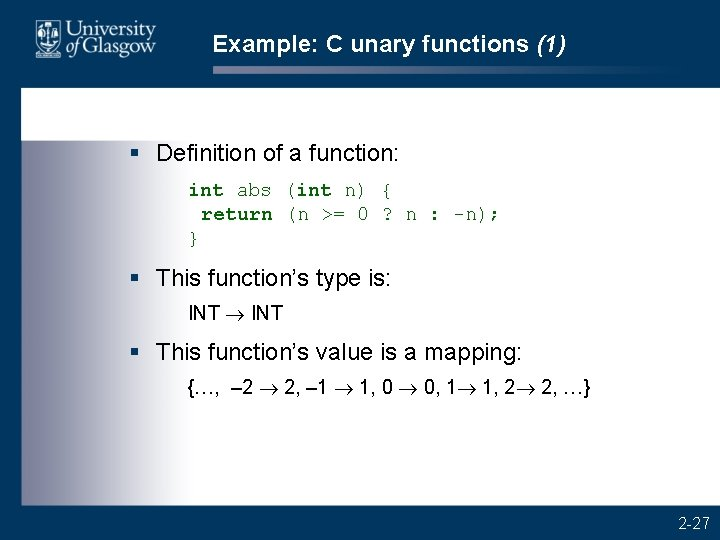Example: C unary functions (1) § Definition of a function: int abs (int n) { return (n >= 0 ? n : -n); } § This function’s type is: INT § This function’s value is a mapping: {…, – 2 2, – 1 1, 0 0, 1 1, 2 2, …} 2 -27Example: C unary functions (2) § Definition of a function: int length (String s) { int n = 0; while (s[n] != NUL) n++; return n; } § This function’s type is: STRING INT § This function’s value is an infinite mapping: {“” 0, “a” 1, “b” 1, “ab” 2, “abc” 3, …} 2 -28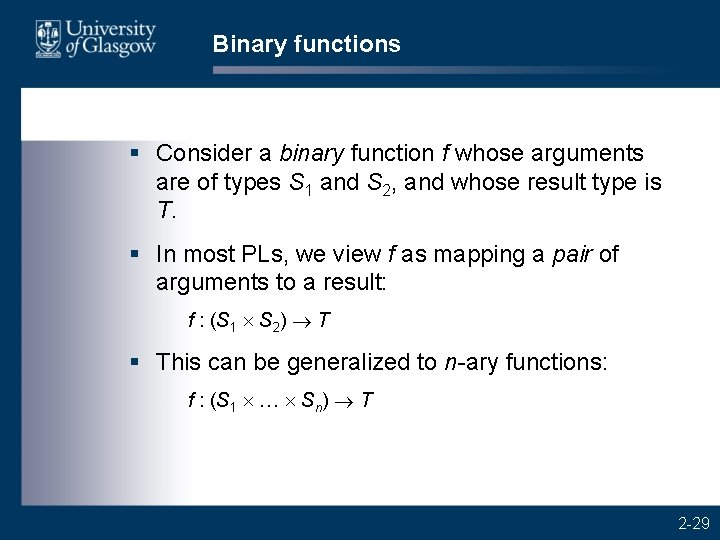Binary functions § Consider a binary function f whose arguments are of types S 1 and S 2, and whose result type is T. § In most PLs, we view f as mapping a pair of arguments to a result: f : (S 1 S 2) T § This can be generalized to n-ary functions: f : (S 1 … Sn) T 2 -29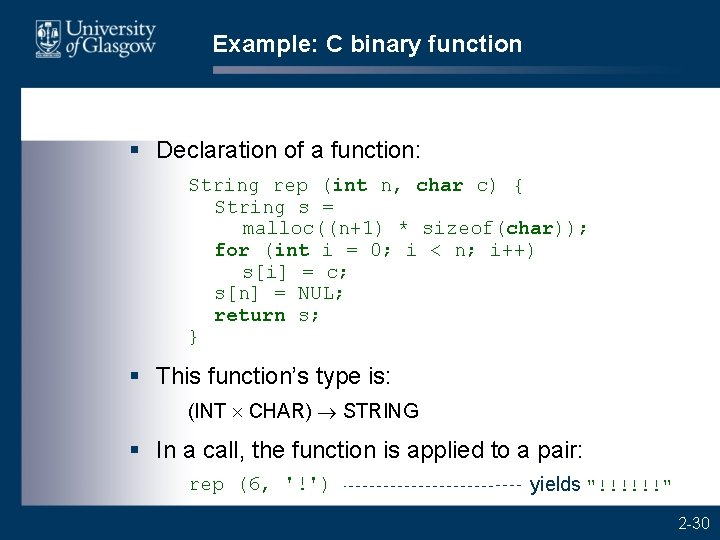Example: C binary function § Declaration of a function: String rep (int n, char c) { String s = malloc((n+1) * sizeof(char)); for (int i = 0; i < n; i++) s[i] = c; s[n] = NUL; return s; } § This function’s type is: (INT CHAR) STRING § In a call, the function is applied to a pair: rep (6, '!') yields "!!!!!!" 2 -30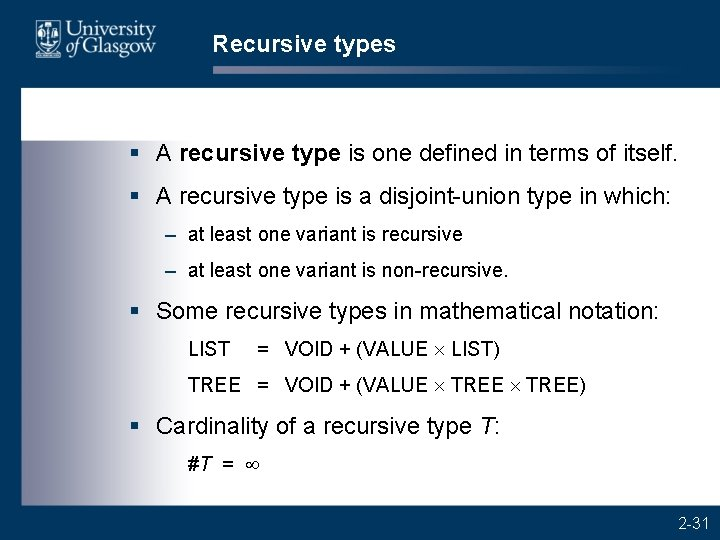Recursive types § A recursive type is one defined in terms of itself. § A recursive type is a disjoint-union type in which: – at least one variant is recursive – at least one variant is non-recursive. § Some recursive types in mathematical notation: LIST = VOID + (VALUE LIST) TREE = VOID + (VALUE TREE) § Cardinality of a recursive type T: #T = 2 -31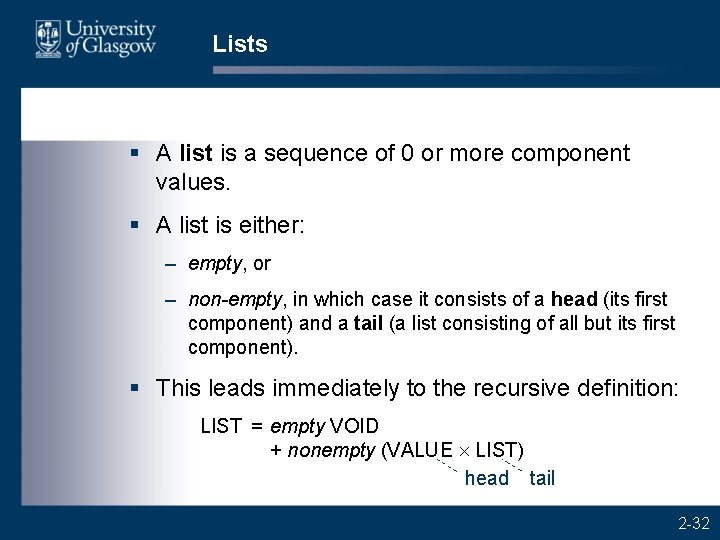Lists § A list is a sequence of 0 or more component values. § A list is either: – empty, or – non-empty, in which case it consists of a head (its first component) and a tail (a list consisting of all but its first component). § This leads immediately to the recursive definition: LIST = empty VOID + nonempty (VALUE LIST) head tail 2 -32Example: Java lists § Class declaration for integer-lists: class Int. List { int head; Int. List tail; } § The non-recursive variant is the built-in null value. 2 -33Strings § A string is a sequence of 0 or more characters. § Python treats strings as primitive. § Haskell treats strings as lists of characters. So strings are equipped with general list operations (head selection, tail selection, concatenation, …). § C treats strings as arrays of characters. So strings are equipped with general array operations (indexing, …). § Java treats strings as objects, of class String. So strings are equipped with the methods of that class. 2 -34Type systems § A type error occurs if a program performs a meaningless operation – such as adding a string to a boolean. § A PL’s type system groups values into types: – to enable programmers to describe data effectively – to help prevent type errors. § Possession of a type system distinguishes highlevel PLs from low-level languages: – In assembly/machine languages, the only “types” are bytes and words, so meaningless operations cannot be prevented. 2 -35Type checking § Before any operation is performed, its operands must be type-checked to prevent a type error. E. g. : – In a not operation, must check that the operand is a boolean. – In an add operation, must check that both operands are numbers. – In an indexing operation, must check that (a) the left operand is an array, and (b) the right operand is an integer. 2 -36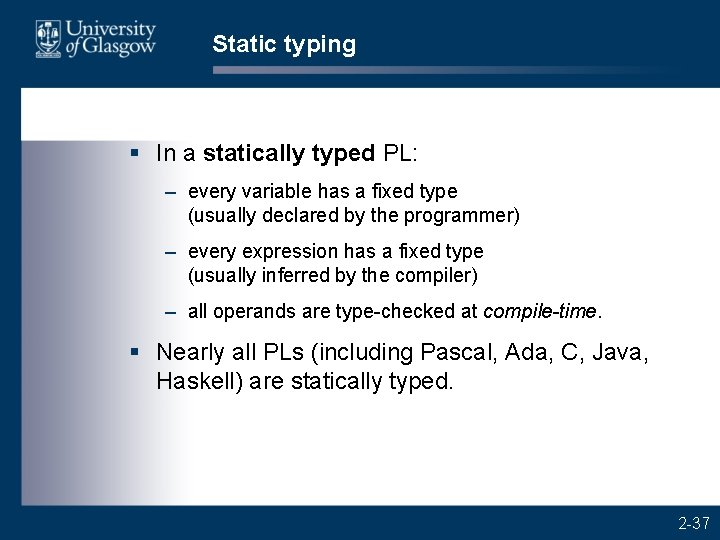Static typing § In a statically typed PL: – every variable has a fixed type (usually declared by the programmer) – every expression has a fixed type (usually inferred by the compiler) – all operands are type-checked at compile-time. § Nearly all PLs (including Pascal, Ada, C, Java, Haskell) are statically typed. 2 -37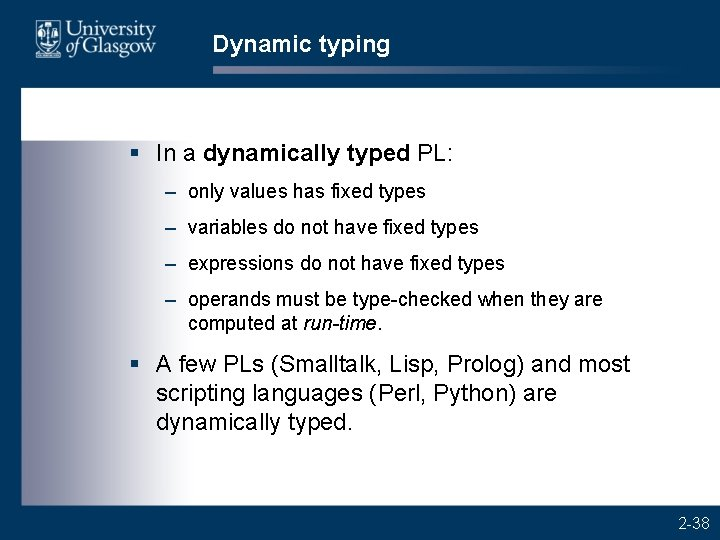Dynamic typing § In a dynamically typed PL: – only values has fixed types – variables do not have fixed types – expressions do not have fixed types – operands must be type-checked when they are computed at run-time. § A few PLs (Smalltalk, Lisp, Prolog) and most scripting languages (Perl, Python) are dynamically typed. 2 -38Example: Java static typing § Java function definition: static boolean even (int n) { return (n%2 == 0); The compiler doesn’t know } n’s value, but does know that § Java function call: int p; … if even(p+1) then … else … n’s type is INT; so it can infer that this expression’s type is BOOL. The compiler doesn’t know p’s value, but does know that p’s type is INT; so it can infer that this expression’s type is INT. This is consistent with the type of even’s parameter. § Even without knowing the values of variables, the Java compiler can guarantee that no type errors will occur at run-time. 2 -39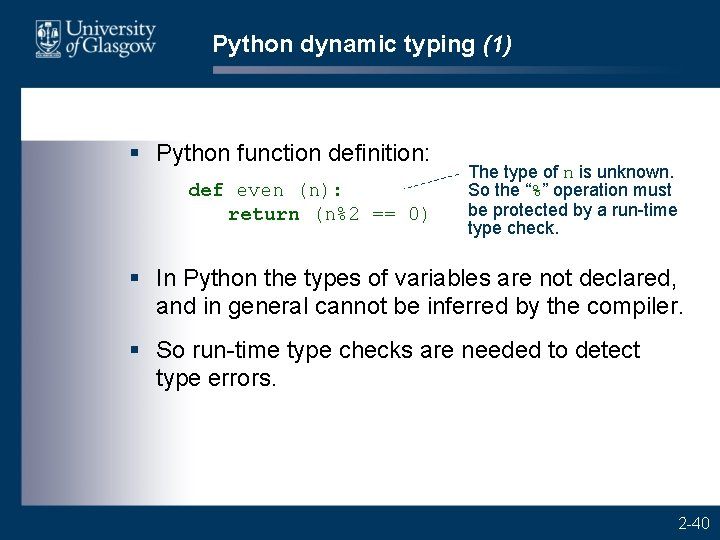Python dynamic typing (1) § Python function definition: def even (n): return (n%2 == 0) The type of n is unknown. So the “%” operation must be protected by a run-time type check. § In Python the types of variables are not declared, and in general cannot be inferred by the compiler. § So run-time type checks are needed to detect type errors. 2 -40Python dynamic typing (2) § Python function definition: def minimum (values): # Return the minimum element of values. min = values which may be for val in values: a tuple or list if val < min: min = val return min 2 -41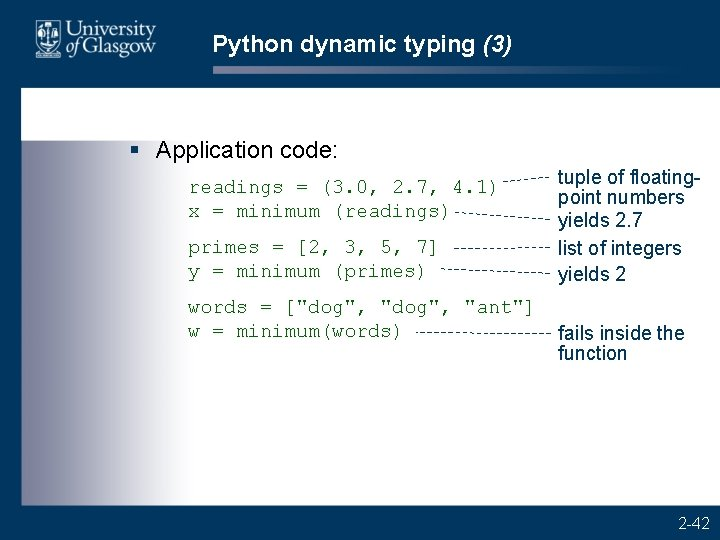Python dynamic typing (3) § Application code: readings = (3. 0, 2. 7, 4. 1) x = minimum (readings) primes = [2, 3, 5, 7] y = minimum (primes) words = ["dog", "ant"] w = minimum(words) tuple of floatingpoint numbers yields 2. 7 list of integers yields 2 fails inside the function 2 -42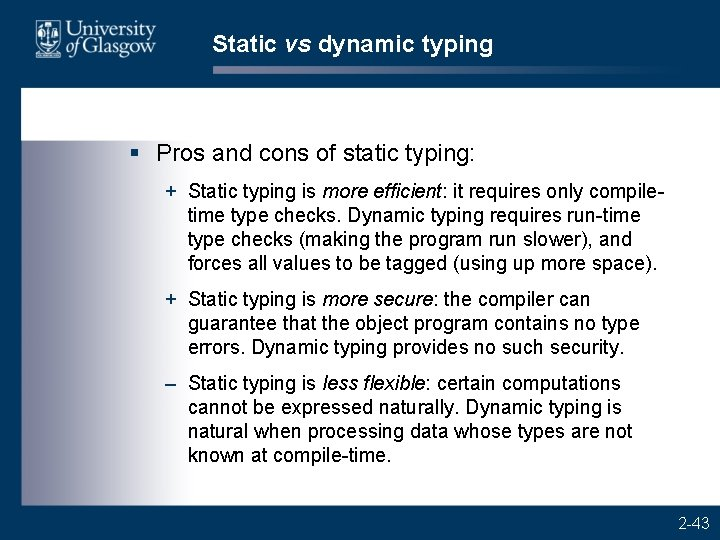Static vs dynamic typing § Pros and cons of static typing: + Static typing is more efficient: it requires only compiletime type checks. Dynamic typing requires run-time type checks (making the program run slower), and forces all values to be tagged (using up more space). + Static typing is more secure: the compiler can guarantee that the object program contains no type errors. Dynamic typing provides no such security. – Static typing is less flexible: certain computations cannot be expressed naturally. Dynamic typing is natural when processing data whose types are not known at compile-time. 2 -43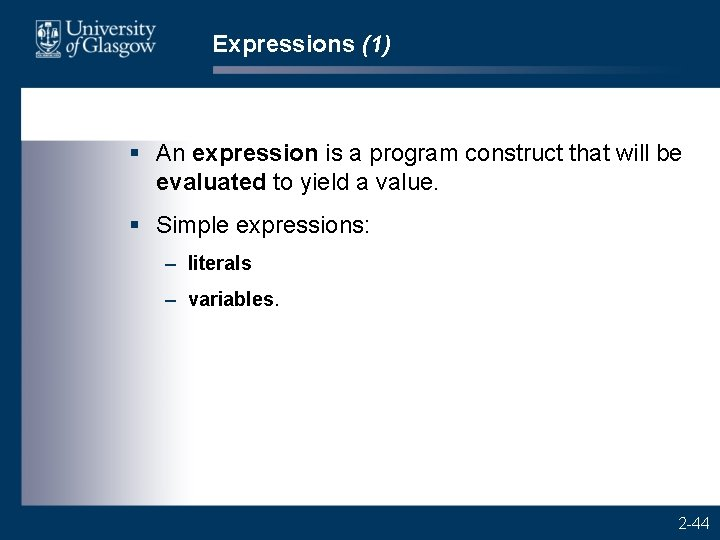Expressions (1) § An expression is a program construct that will be evaluated to yield a value. § Simple expressions: – literals – variables. 2 -44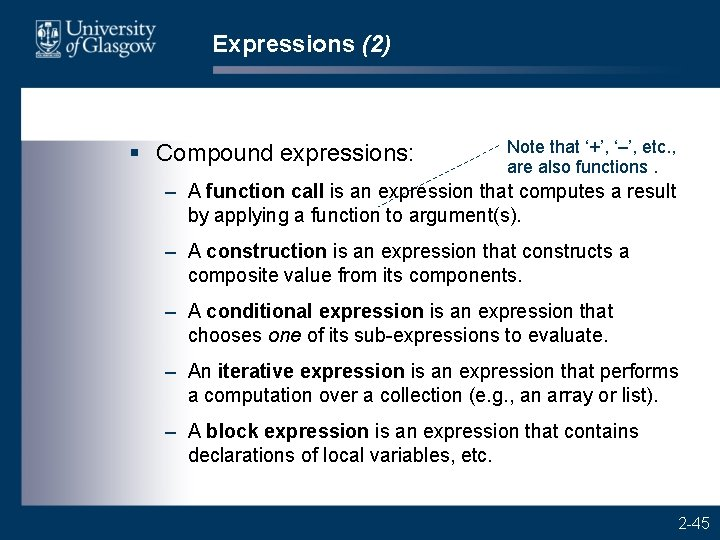Expressions (2) § Compound expressions: Note that ‘+’, ‘–’, etc. , are also functions. – A function call is an expression that computes a result by applying a function to argument(s). – A construction is an expression that constructs a composite value from its components. – A conditional expression is an expression that chooses one of its sub-expressions to evaluate. – An iterative expression is an expression that performs a computation over a collection (e. g. , an array or list). – A block expression is an expression that contains declarations of local variables, etc. 2 -45Example: constructions § Python tuple and list constructions: new. Years. Day = ("JAN", 1) tomorrow = (m, d+1) primes = [2, 3, 5, 7, 11] size = [31, 29 if is. Leap(y) else 28, 31, 30, 31] § Java array and object constructions: int[] primes = {2, 3, 5, 7, 11}; Date new. Years. Day = new Date(JAN, 1); Date tomorrow = new Date(m, d+1); 2 -46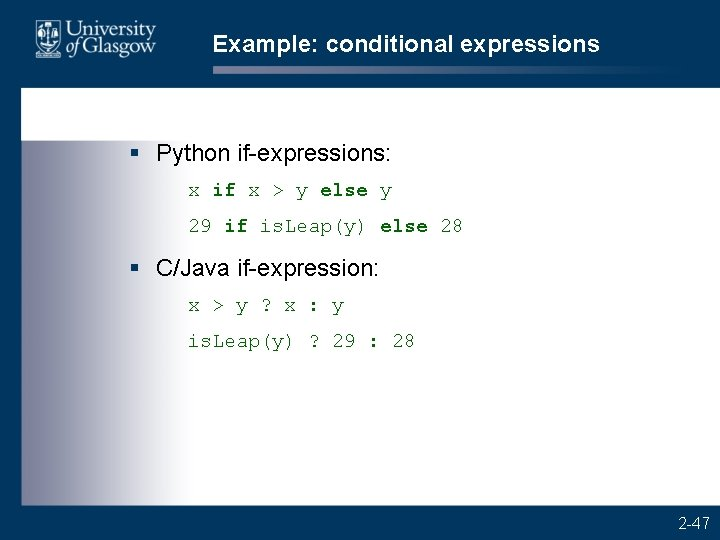Example: conditional expressions § Python if-expressions: x if x > y else y 29 if is. Leap(y) else 28 § C/Java if-expression: x > y ? x : y is. Leap(y) ? 29 : 28 2 -47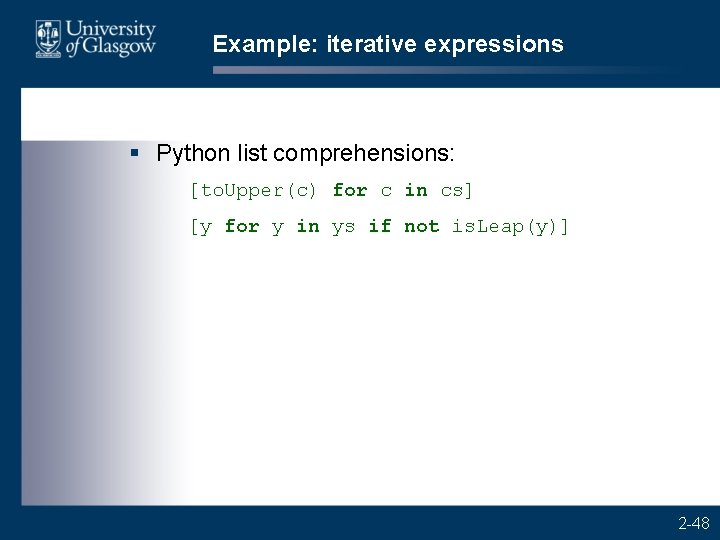Example: iterative expressions § Python list comprehensions: [to. Upper(c) for c in cs] [y for y in ys if not is. Leap(y)] 2 -48